# TableBased

Incompressible medium properties based on tables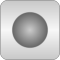# Package Contents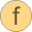invertTemp Function to invert temperatures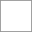BaseProperties Base properties of T dependent mediumsetState_pTX Returns state record, given pressure and temperaturesetState_dTX Returns state record, given pressure and temperaturesetState_pT Returns state record as function of p and TsetState_phX Returns state record, given pressure and specific enthalpysetState_ph Returns state record as function of p and hsetState_psX Returns state record, given pressure and specific entropysetState_ps Returns state record as function of p and ssetSmoothState Return thermodynamic state so that it smoothly approximates: if x > 0 then state_a else state_bspecificHeatCapacityCv Specific heat capacity at constant volume (or pressure) of mediumspecificHeatCapacityCp Specific heat capacity at constant volume (or pressure) of mediumdynamicViscosity Return dynamic viscosity as a function of the thermodynamic state recordthermalConductivity Return thermal conductivity as a function of the thermodynamic state records_T Compute specific entropyspecificEntropy Return specific entropy as a function of the thermodynamic state recordh_T Compute specific enthalpy from temperatureh_T_der Compute specific enthalpy from temperatureh_pT Compute specific enthalpy from pressure and temperaturedensity_T Return density as function of temperaturetemperature Return temperature as a function of the thermodynamic state recordpressure Return pressure as a function of the thermodynamic state recorddensity Return density as a function of the thermodynamic state recordspecificEnthalpy Return specific enthalpy as a function of the thermodynamic state recordspecificInternalEnergy Return specific internal energy as a function of the thermodynamic state recordT_ph Compute temperature from pressure and specific enthalpyT_ps Compute temperature from pressure and specific enthalpy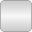Polynomials_Temp Temporary Functions operating on polynomials (including polynomial fitting); only to be used in Modelica.Media.Incompressible.TableBased

# Package Constants (51)

ThermoStates Value: if enthalpyOfT then Modelica.Media.Interfaces.Choices.IndependentVariables.T else Modelica.Media.Interfaces.Choices.IndependentVariables.pT Type: IndependentVariables Description: Enumeration type for independent variables Value: "tableMedium" Type: String Description: Name of the medium Value: {mediumName} Type: String[:] Description: Names of the mixture substances. Set substanceNames={mediumName} if only one substance. Value: fill("", 0) Type: String[:] Description: Names of the additional (extra) transported properties. Set extraPropertiesNames=fill("",0) if unused Value: true Type: Boolean Description: = true, if u and d are not a function of pressure Value: true Type: Boolean Description: = true if medium contains the equation sum(X) = 1.0; set reducedX=true if only one substance (see docu for details) Value: true Type: Boolean Description: = true if medium contains the equation X = reference_X Value: 1.013e5 Type: AbsolutePressure (Pa) Description: Reference pressure of Medium: default 1 atmosphere Value: 298.15 Type: Temperature (K) Description: Reference temperature of Medium: default 25 deg Celsius Value: fill(1 / nX, nX) Type: MassFraction[nX] (kg/kg) Description: Default mass fractions of medium Value: 101325 Type: AbsolutePressure (Pa) Description: Default value for pressure of medium (for initialization) Value: Modelica.SIunits.Conversions.from_degC(20) Type: Temperature (K) Description: Default value for temperature of medium (for initialization) Value: specificEnthalpy_pTX(p_default, T_default, X_default) Type: SpecificEnthalpy (J/kg) Description: Default value for specific enthalpy of medium (for initialization) Value: reference_X Type: MassFraction[nX] (kg/kg) Description: Default value for mass fractions of medium (for initialization) Value: fill(0, nC) Type: ExtraProperty[nC] Description: Default value for trace substances of medium (for initialization) Value: size(substanceNames, 1) Type: Integer Description: Number of substances Value: nS Type: Integer Description: Number of mass fractions Value: if fixedX then 0 else if reducedX then nS - 1 else nS Type: Integer Description: Number of structurally independent mass fractions (see docu for details) Value: size(extraPropertiesNames, 1) Type: Integer Description: Number of extra (outside of standard mass-balance) transported properties Value: 1.0e-6 * ones(nC) Type: Real[nC] Description: Default for the nominal values for the extra properties Value: true Type: Boolean Description: True if enthalpy is approximated as a function of T only, (p-dependence neglected) Value: size(tableDensity, 1) > 1 Type: Boolean Description: True if density is a function of temperature Value: Type: Temperature (K) Description: Minimum temperature valid for medium model Value: Type: Temperature (K) Description: Maximum temperature valid for medium model Value: 273.15 Type: Temperature (K) Description: Reference Temperature Value: 0 Type: SpecificEnthalpy (J/kg) Description: Reference enthalpy at T0, reference_p Value: 0 Type: SpecificEntropy (J/(kg·K)) Description: Reference entropy at T0, reference_p Value: 0.1 Type: MolarMass (kg/mol) Description: Molar mass Value: 2 Type: Integer Description: Degree of polynomial used for fitting Value: npol Type: Integer Description: Degree of polynomial used for fitting rho(T) Value: npol Type: Integer Description: Degree of polynomial used for fitting Cp(T) Value: npol Type: Integer Description: Degree of polynomial used for fitting eta(T) Value: npol Type: Integer Description: Degree of polynomial used for fitting pVap(T) Value: npol Type: Integer Description: Degree of polynomial used for fitting lambda(T) Value: size(tableViscosity, 1) Type: Integer Description: Number of data points for viscosity Value: Type: Real[:,2] Description: Table for rho(T) Value: Type: Real[:,2] Description: Table for Cp(T) Value: Type: Real[:,2] Description: Table for eta(T) Value: Type: Real[:,2] Description: Table for pVap(T) Value: Type: Real[:,2] Description: Table for lambda(T) Value: Type: Boolean Description: True if T[K],Kelvin used for table temperatures Value: not size(tableDensity, 1) == 0 Type: Boolean Description: True if table tableDensity is present Value: not size(tableHeatCapacity, 1) == 0 Type: Boolean Description: True if table tableHeatCapacity is present Value: not size(tableViscosity, 1) == 0 Type: Boolean Description: True if table tableViscosity is present Value: not size(tableVaporPressure, 1) == 0 Type: Boolean Description: True if table tableVaporPressure is present Value: if size(tableViscosity, 1) > 0 then if TinK then 1 ./ tableViscosity[:, 1] else 1 ./ Cv.from_degC(tableViscosity[:, 1]) else fill(0, neta) Type: Real[neta] Value: if hasDensity then Poly.fitting(tableDensity[:, 1], tableDensity[:, 2], npolDensity) else zeros(npolDensity + 1) Type: Real[:] Value: if hasHeatCapacity then Poly.fitting(tableHeatCapacity[:, 1], tableHeatCapacity[:, 2], npolHeatCapacity) else zeros(npolHeatCapacity + 1) Type: Real[:] Value: if hasViscosity then Poly.fitting(invTK, Math.log(tableViscosity[:, 2]), npolViscosity) else zeros(npolViscosity + 1) Type: Real[:] Value: if hasVaporPressure then Poly.fitting(tableVaporPressure[:, 1], tableVaporPressure[:, 2], npolVaporPressure) else zeros(npolVaporPressure + 1) Type: Real[:] Value: if size(tableConductivity, 1) > 0 then Poly.fitting(tableConductivity[:, 1], tableConductivity[:, 2], npolConductivity) else zeros(npolConductivity + 1) Type: Real[:]

# Information

This information is part of the Modelica Standard Library maintained by the Modelica Association.

This is the base package for medium models of incompressible fluids based on tables. The minimal data to provide for a useful medium description is tables of density and heat capacity as functions of temperature.

It should be noted that incompressible media only have 1 state per control volume (usually T), but have both T and p as inputs for fully correct properties. The error of using only T-dependent properties is small, therefore a Boolean flag enthalpyOfT exists. If it is true, the enumeration Choices.independentVariables is set to Choices.independentVariables.T otherwise it is set to Choices.independentVariables.pT.

#### Using the package TableBased

To implement a new medium model, create a package that extends TableBased and provides one or more of the constant tables:

```tableDensity        = [T, d];
tableHeatCapacity   = [T, Cp];
tableConductivity   = [T, lam];
tableViscosity      = [T, eta];
tableVaporPressure  = [T, pVap];
```

The table data is used to fit constant polynomials of order npol, the temperature data points do not need to be same for different properties. Properties like enthalpy, inner energy and entropy are calculated consistently from integrals and derivatives of d(T) and Cp(T). The minimal data for a useful medium model is thus density and heat capacity. Transport properties and vapor pressure are optional, if the data tables are empty the corresponding function calls can not be used.

# Extended by (2)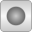Essotherm650 Modelica.Media.Incompressible.Examples Essotherm thermal oilGlycol47 Modelica.Media.Incompressible.Examples 1,2-Propylene glycol, 47% mixture with water